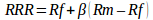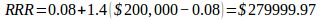# How to Calculate Required Rate of Return?Image credit: RawPixel

The required rate of return (RRR) is the minimum return an investor is ready to accept on investment, and play a major role in driving securities prices in the financial markets. This is one of many metrics and calculations used in corporate finance and equity valuation. RRR goes beyond just pinpointing the return of investment as it factors in risk as a key consideration in determining potential return. It is a key aspect in dealing with finance problems. This rate is meant to compensate the investor for the riskiness of the investment, and if the expected return on investment doesn’t meet or exceeds the RRR, then the investor won’t invest.The Capital Asset Pricing Model (CAPM) technique is used in calculating RRR. To do this, three components must be considered; the average market return, the beta, and the rate of return on a risk-free investment. The required rate of return can also be estimated by finding the cost of equity of investments or projects with similar risk. For instance, if a business has several sources of equity—like preferred stock and common stock—then the cost of equity will be weighed on different return rates. Some analysts use a rate calculator to calculate RRR. But, in this post, we will look at each and every metric in details and show you how to determine required rate of return equation and present value annuity formula.

##Where:

• RRR is the Required Rate of Return
• RF is the Risk-Free Rate
• β is the Beta
• Rm is the Market Return

Calculating the required rate of return appears more complex than they actually are. Below, we provide a formula for required rate of return to help you understand how it works. If you are provided a company’s expected return of \$200, 000, a risk coefficient (β) of 1.4, and a risk-free rate of 8%, then you’ll work out the calculation as follows;

Using the formula above, the RF is 8%, β 1.4, and expected return \$ 200, 000. Therefore;## How to Find Required Rate of Return for Equity

A stock’s RRR on equity calculates the expected return on how risky the stock is as an investment. The beta value compares the business’s overall market and is used to offer the riskiness of the stock. Normally, the beta value in the market is 1, any value that is greater than this would mean that it is riskier and should, therefore, offer a higher return to reimburse this additional risk.

Use the following steps to determine the required rate of return for equity:

• Start by deducting the risk-free rate from the market rate to get percentage market premium. For example, if the overall market offers and 10% return, and you wish to invest 5%, then subtract this value from 10% to get 5%, or 0.05.
• Secondly, get a beta value. In most cases, the beta value for a stock can be found on most finance and investment websites. Just look up for this value in websites such as cnbc.com and money.msn.com. For this post, let’s assume the beta is 1.25.
• Multiply the resulting figure in step 1 above (0.05) with the beta value to give you the market risk premium. In our example, you will have 0.063.
• Finally, to obtain the required rate of return on equity, add the risk-free rate to the market risk premium. From our example, 0.063 + 0.05 = 0.113. Therefore, your stock would need to offer 0.113 or 11.3% return on equity to be worth the risk associated with the stock.

## What Problems Do You Encounter When Calculating Required Rate of Return and Why You Need Our Help?

Calculating the required rate of return helps you in financial forecasting. RRR not only indicates the return of investment, but it also shows the risks associated the potential return. So, if you ever wondered what is forecasting in finance, this right here is an example. However, sometimes problems arise when trying to find a suitable proxy beta—betas vary with websites. Another difficulty arises when you are told to calculate the required rate of return using other methods other than the CAPM. And just like we’ve shown you how to calculate RRR using CAPM method and how to calculate the same for equity we can also help you work out assignments that require other approaches such as the Discount Divided Approach. Besides helping you work out finance problems, we also help you with the mastery of confusing formulas such as bond valuation formula and present value annuity formula, among others.

We also offer a helping hand in assignments that require finding required rate of return for corporate finance. If you wish to seek our professional help, we will be glad to help at a low cost. You can post your queries on our ever present 24/7 chat tool, and our professionals in business, economics, and finance will attend to your queries through and through. We also promise our esteemed clients quality and original work which is delivered in real time.